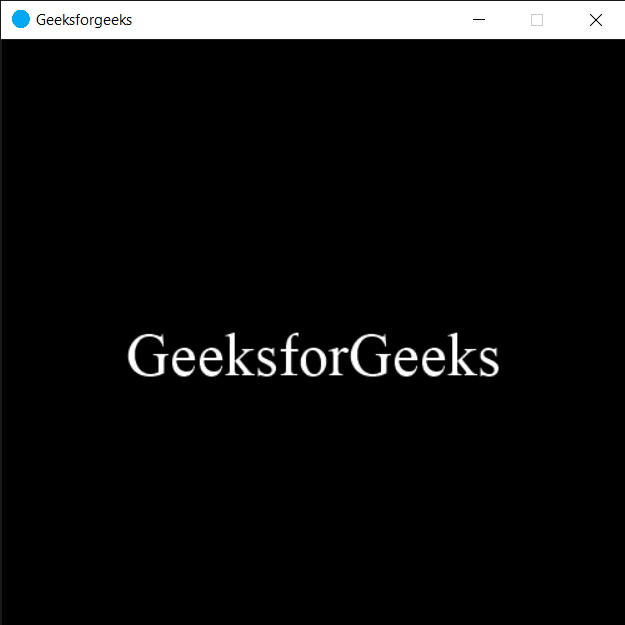Related Articles
PYGLET – On Show Event
• Last Updated : 22 Sep, 2020

In this article we will see how we can trigger on show event in PYGLET module in python. Pyglet is easy to use but powerful library for developing visually rich GUI applications like games, multimedia etc. A window is a “heavyweight” object occupying operating system resources. Windows may appear as floating regions or can be set to fill an entire screen (fullscreen).This event is triggered when a window is restored after being minimised, or after being displayed for the first time.

We can create a window with the help of command given below

```pyglet.window.Window(width, height, title)
```

Below is the syntax of the on show event, this method get called when this event is triggered

```@window.event
def on_show():
print("Window is shown")
```

Below is the implementation

 `# importing pyglet module ` `import` `pyglet ` `import` `pyglet.window.key ` ` `  `# width of window ` `width ``=` `500` ` `  `# height of window ` `height ``=` `500` ` `  `# caption i.e title of the window ` `title ``=` `"Geeksforgeeks"` ` `  `# creating a window ` `window ``=` `pyglet.window.Window(width, height, title) ` ` `  `# text  ` `text ``=` `"GeeksforGeeks"` ` `  `# creating a label with font = times roman ` `# font size = 36 ` `# aligning it to the centre ` `label ``=` `pyglet.text.Label(text, ` `                          ``font_name ``=``'Times New Roman'``, ` `                          ``font_size ``=` `36``, ` `                          ``x ``=` `window.width``/``/``2``, y ``=` `window.height``/``/``2``, ` `                          ``anchor_x ``=``'center'``, anchor_y ``=``'center'``) ` ` `  `new_label ``=` `pyglet.text.Label(text, ` `                          ``font_name ``=``'Times New Roman'``, ` `                          ``font_size ``=` `10``, ` `                          ``x ``=` `25``, y ``=` `25``) ` ` `  `# on draw event ` `@window``.event ` `def` `on_draw():     ` ` `  `     `  `    ``# clearing the window ` `    ``window.clear() ` `     `  `    ``# drawing the label on the window ` `    ``label.draw() ` ` `  `     `  `# key press event     ` `@window``.event ` `def` `on_key_press(symbol, modifier): ` ` `  `    ``# key "C" get press ` `    ``if` `symbol ``=``=` `pyglet.window.key.C: ` `         `  `        ``print``(``"Key C is pressed"``) ` ` `  ` `  `# on show event ` `@window``.event ` `def` `on_show(): ` `     `  `    ``# prinitng some message ` `    ``print``(``"Window is displayed"``) ` ` `  `# image for icon ` `img ``=` `image ``=` `pyglet.resource.image(``"logo.png"``) ` ` `  `# setting image as icon ` `window.set_icon(img) ` `                `  `# start running the application ` `pyglet.app.run() `

Output :```Window is displayed
```

Attention geek! Strengthen your foundations with the Python Programming Foundation Course and learn the basics.

To begin with, your interview preparations Enhance your Data Structures concepts with the Python DS Course.

My Personal Notes arrow_drop_up
Recommended Articles
Page :